06 325 750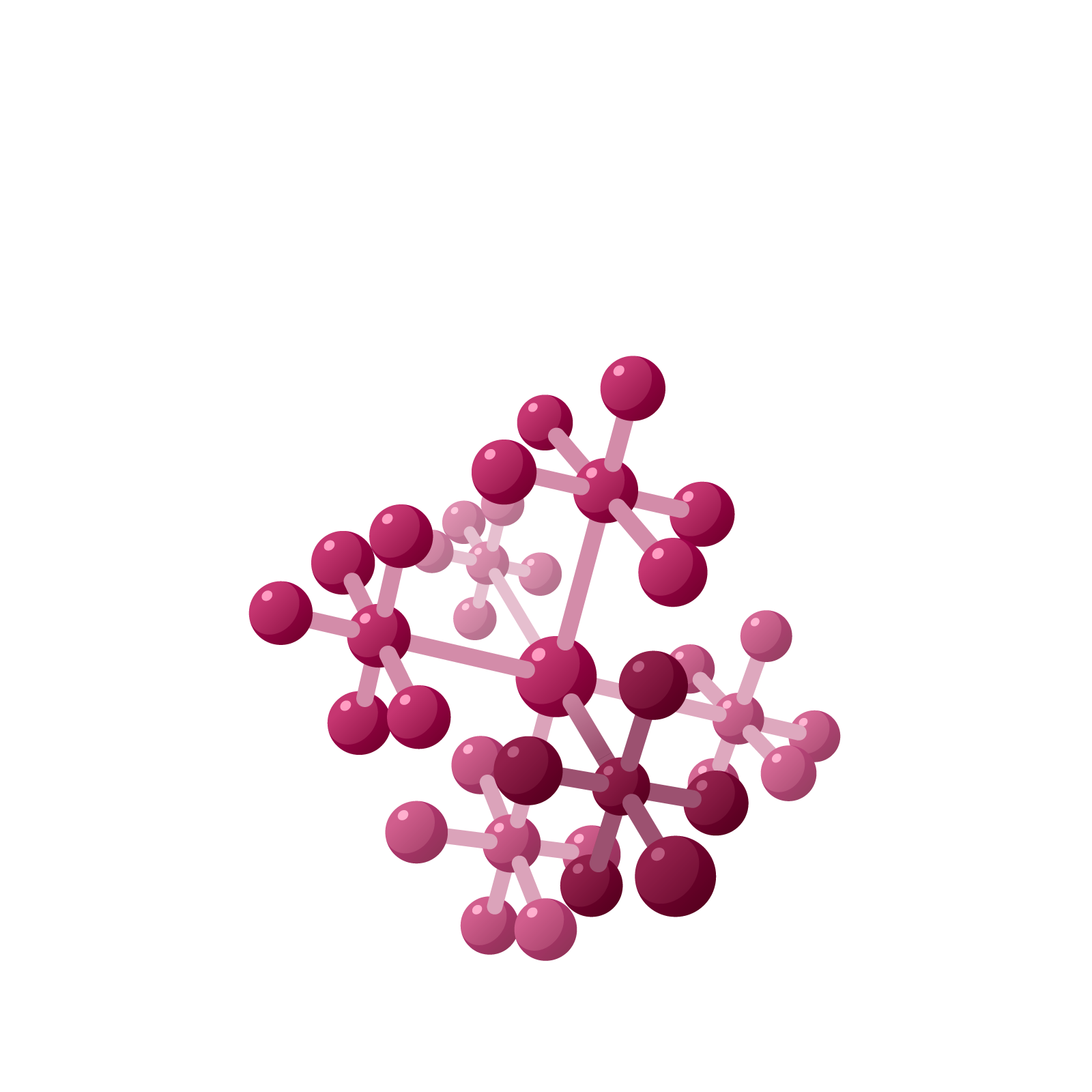# Station  11FDivisionEarlier we computed $19 \times 5$ and got this picture for the answer $95$.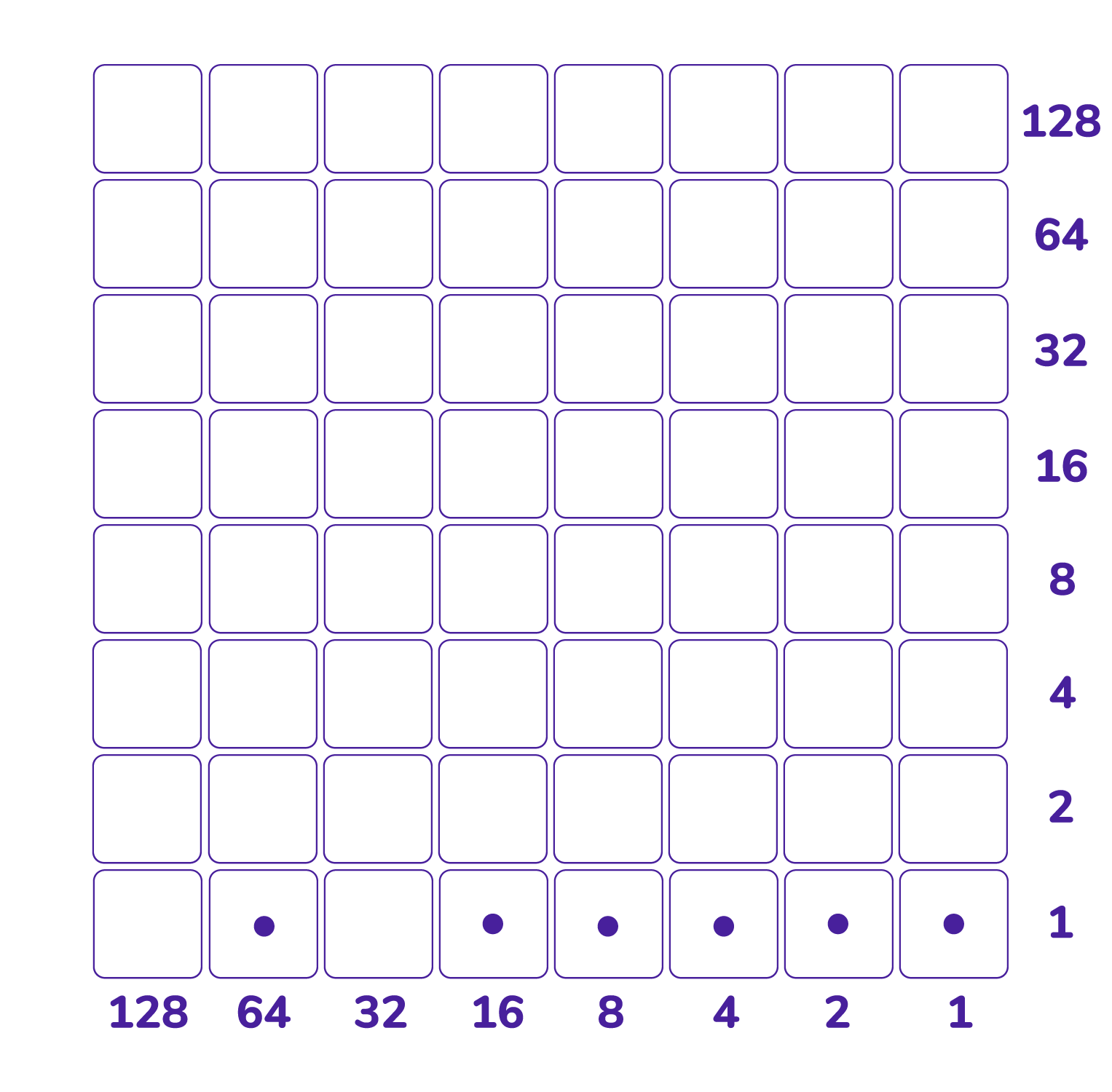If we were given this picture of $95$ first and was told that it came from a multiplication problem with one of the factors being $5$, could we deduce what the other factor must have been? That is, can we use the picture to compute $95 \div 5$?Since $5 = 4 + 1$ we will need to slide counters on this picture so that two copies of the same pattern appear in the shaded two rows.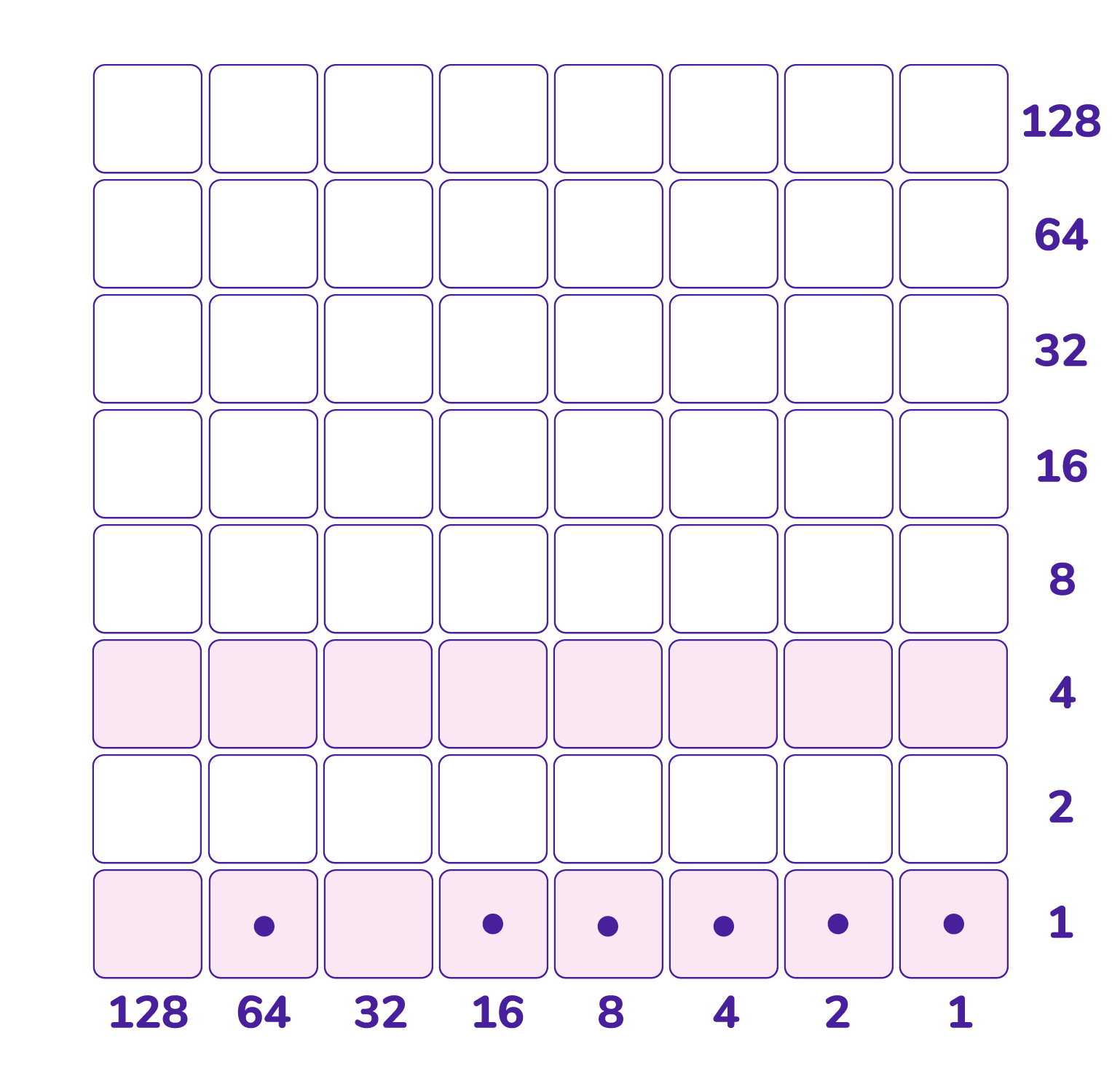Slide the leftmost dot up to the top shaded row and we see it “completes” the $16$ column. Let’s not touch the counters in that column ever again.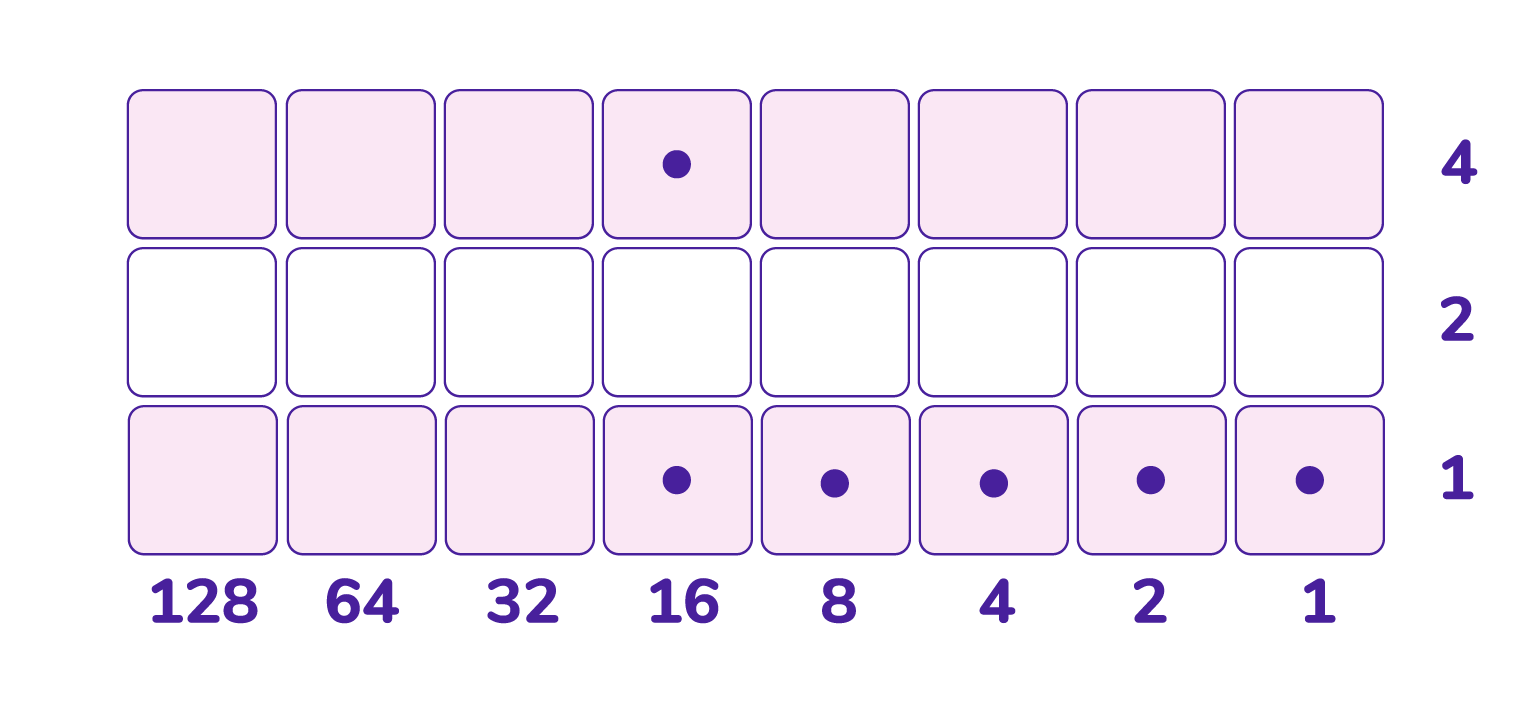We are now left with a smaller division problem: dividing $8+4+2+1$ (that is, $15$) by $5$.

Slide its leftmost dot up to the top shaded row. This completes the $2$s column and let’s never touch the counters in that column again.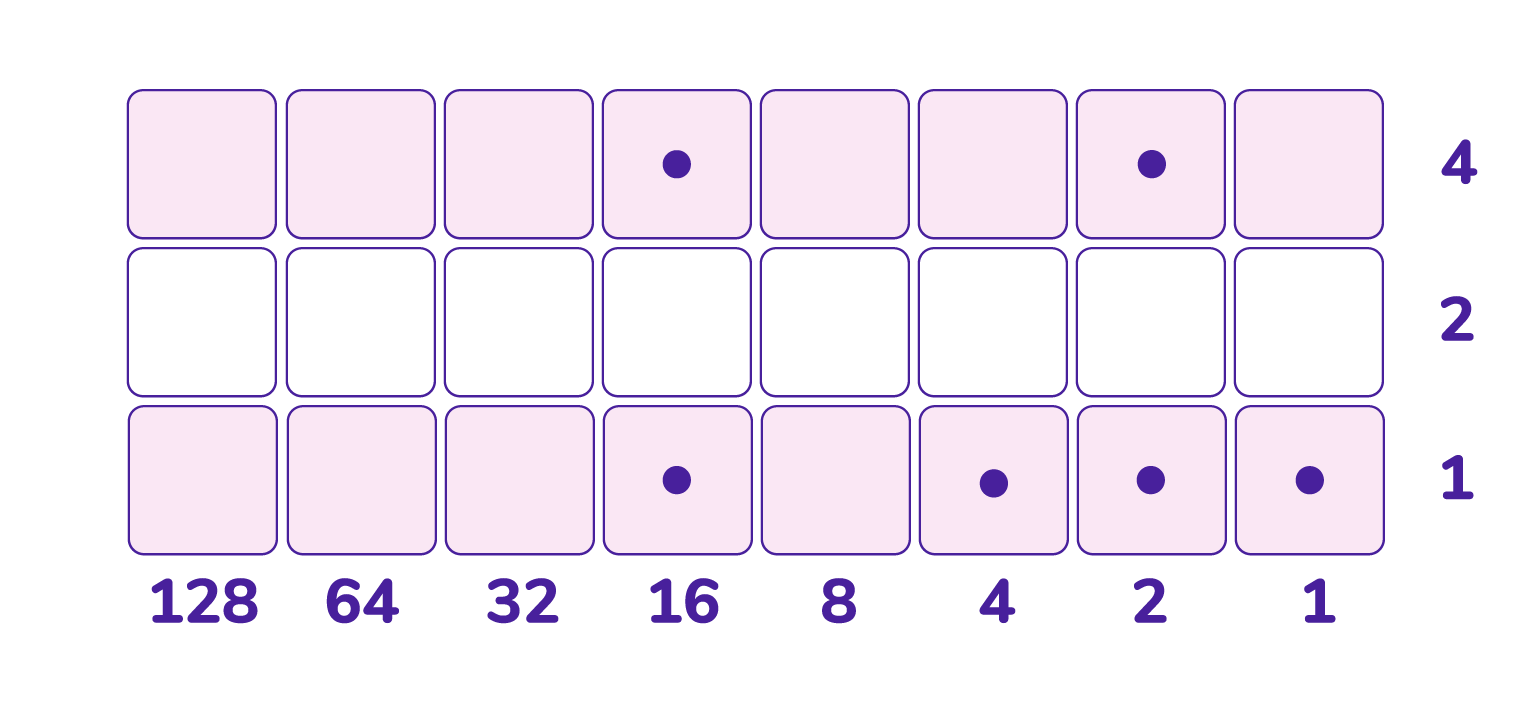This leaves us with a smaller division problem to contend with: $4+1$ divided by $5$. Slide its leftmost dot up to the top shaded row to complete the $1$s column.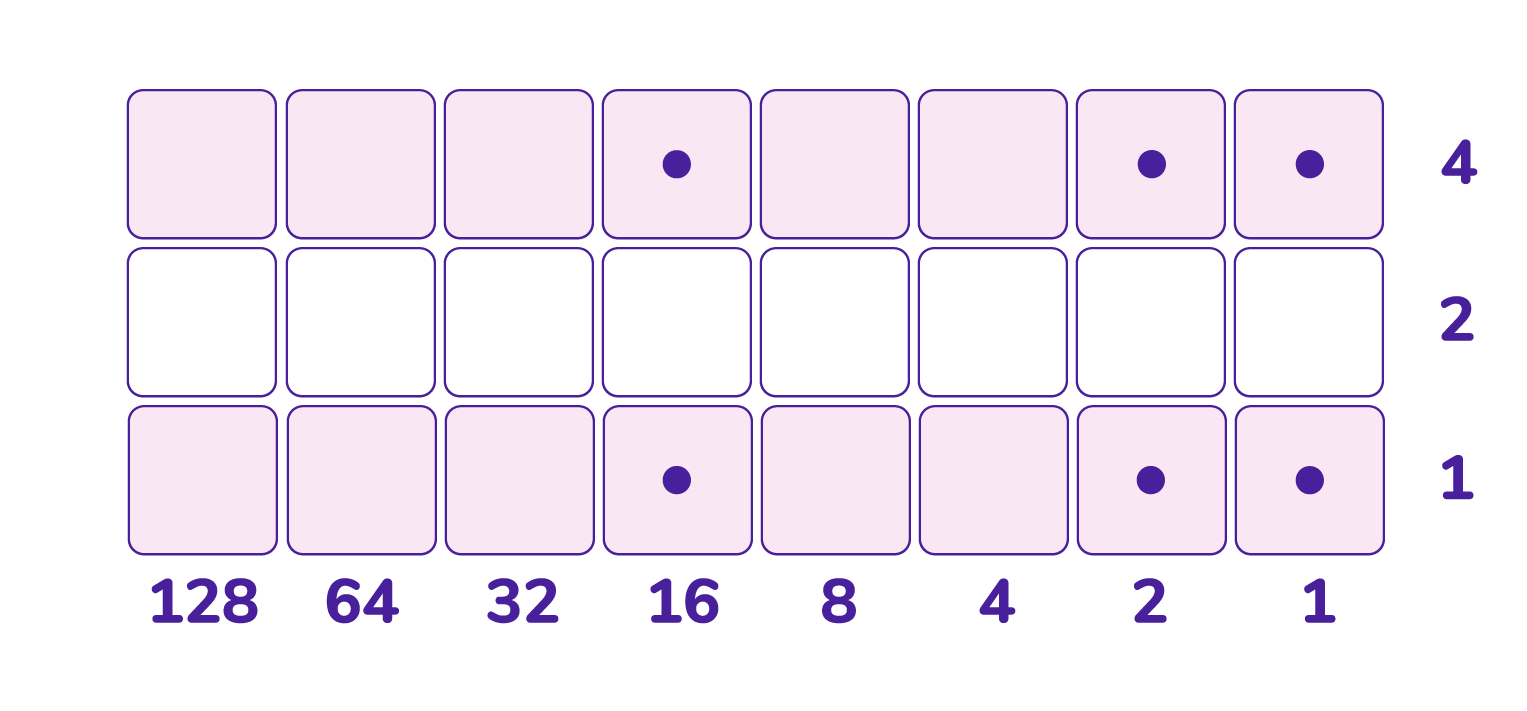We see that we have now created the picture of $19 \times 5$ and so $95 \div 5 = 19$.

This loosely illustrates the general principle for doing division on Napier’s checkerboard:

Represent the dividend by dots in the bottom row and the divisor by shaded rows.

Slide the leftmost dot to the top shaded row.

Complete the leftmost column of dots possible in some way you can (you might need to unexplode some dots) and when done never touch those dots again. What is left is a smaller division problem and repeat this procedure for the leftmost dot of that problem.

The procedure described here is loose as our computation $95 \div 5 = 19$ ran into no difficulties.

Let’s try $250 \div 13$ for something more involved. Here’s its setup.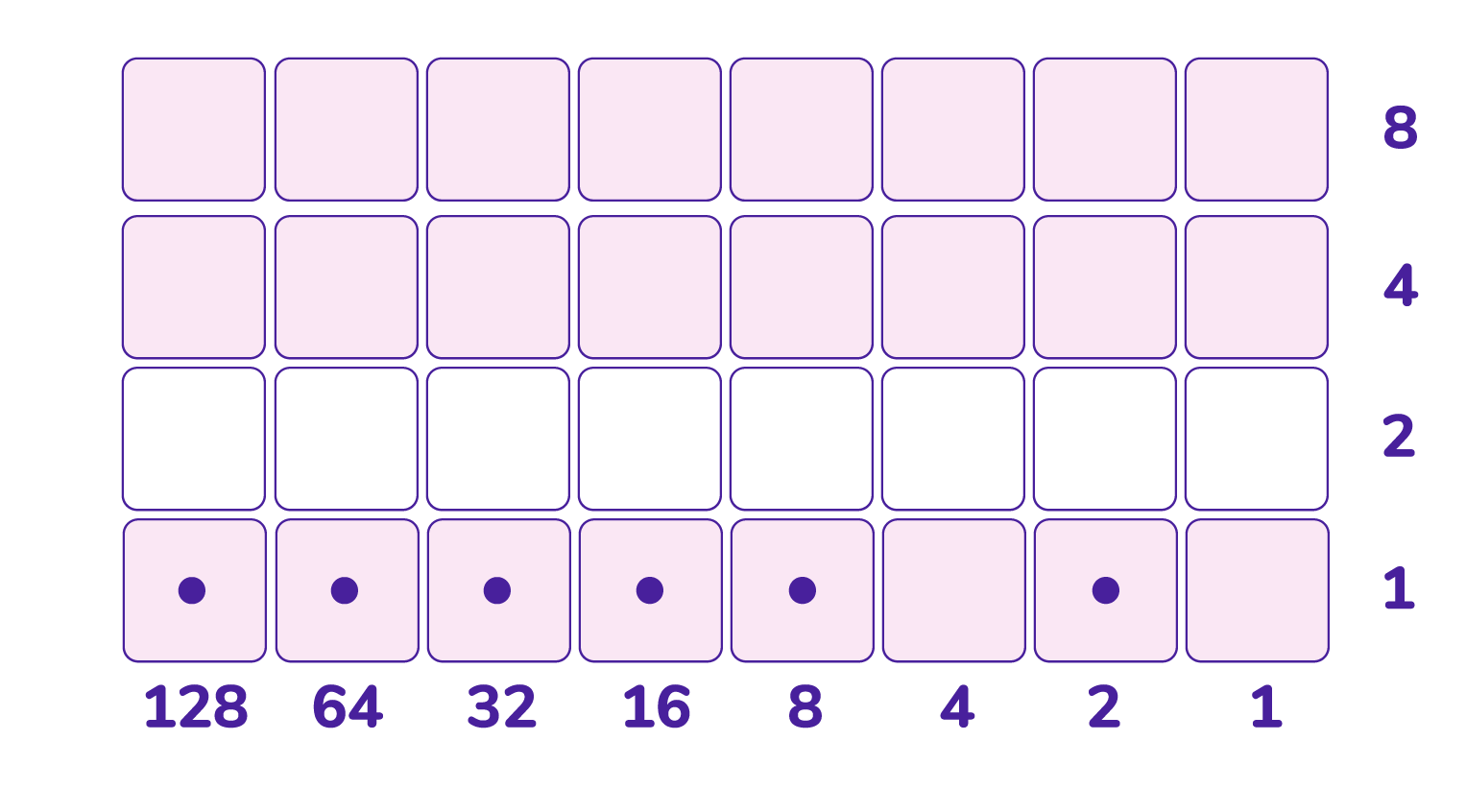Slide the leftmost dot to the highest shaded row. Doing so shows we need to work with the $16$s column, but it is not complete.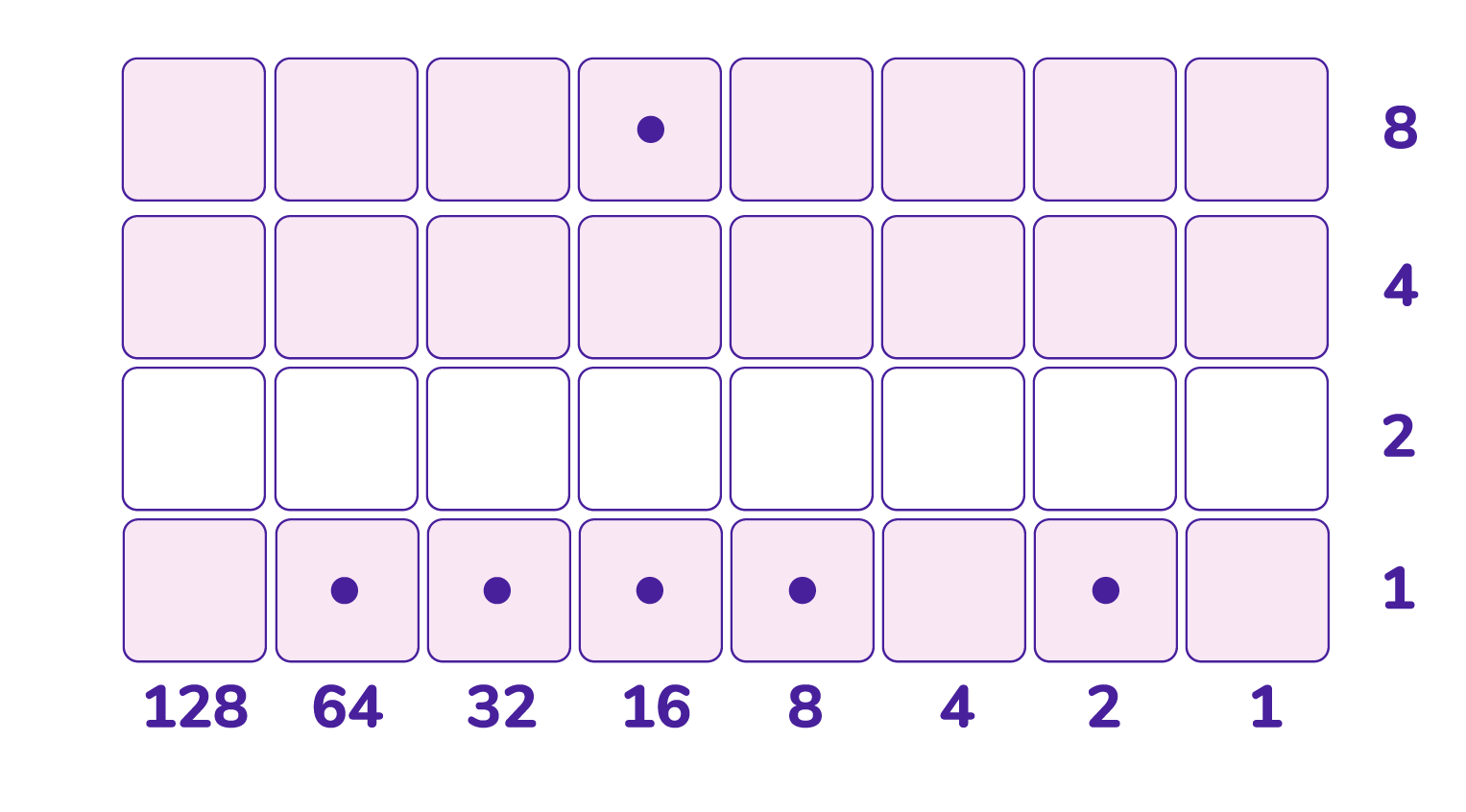We can complete it by sliding the current leftmost dot into that column. (That’s convenient!)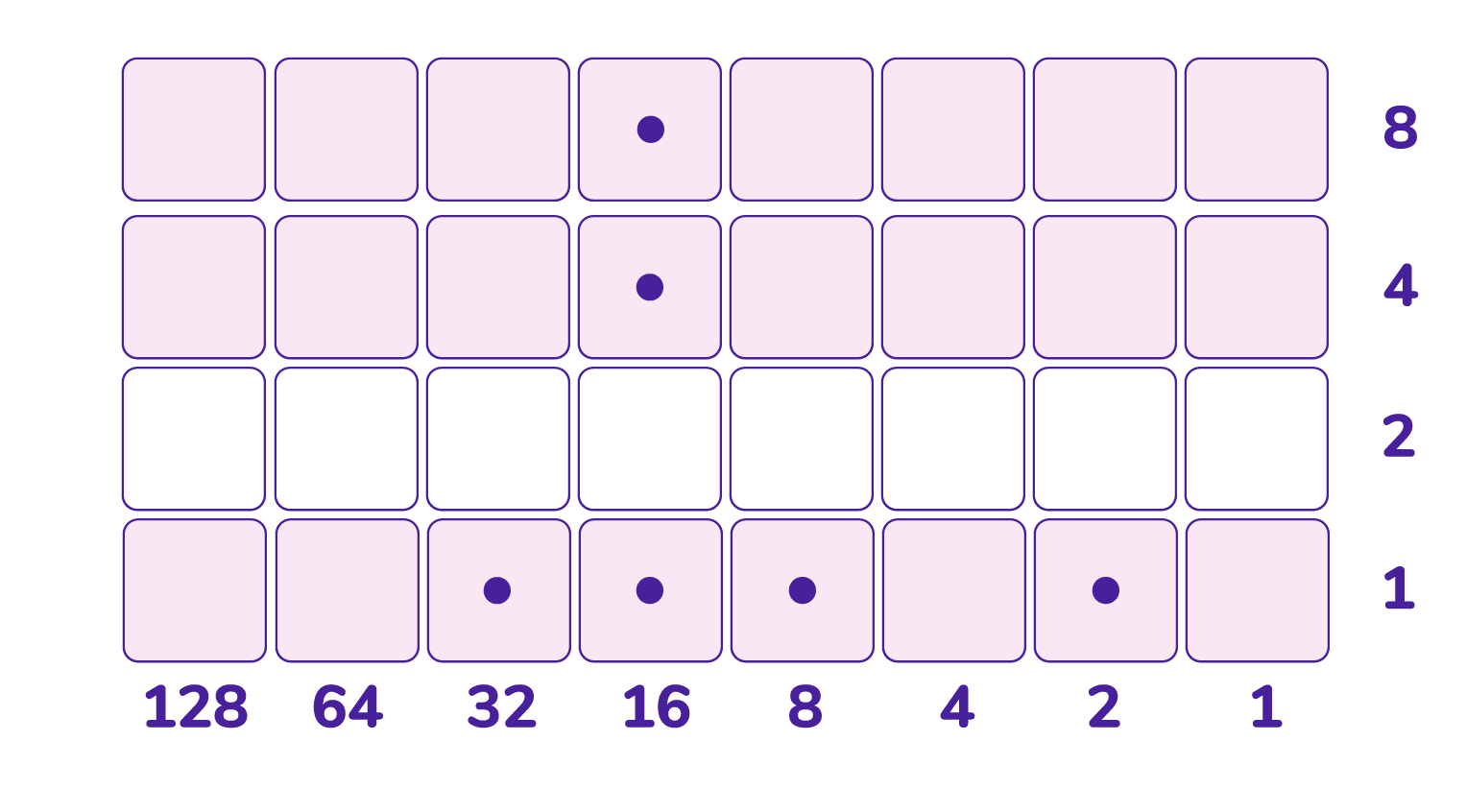Now we have a smaller division problem to work on. Slide the leftmost dot up to the highest shaded row.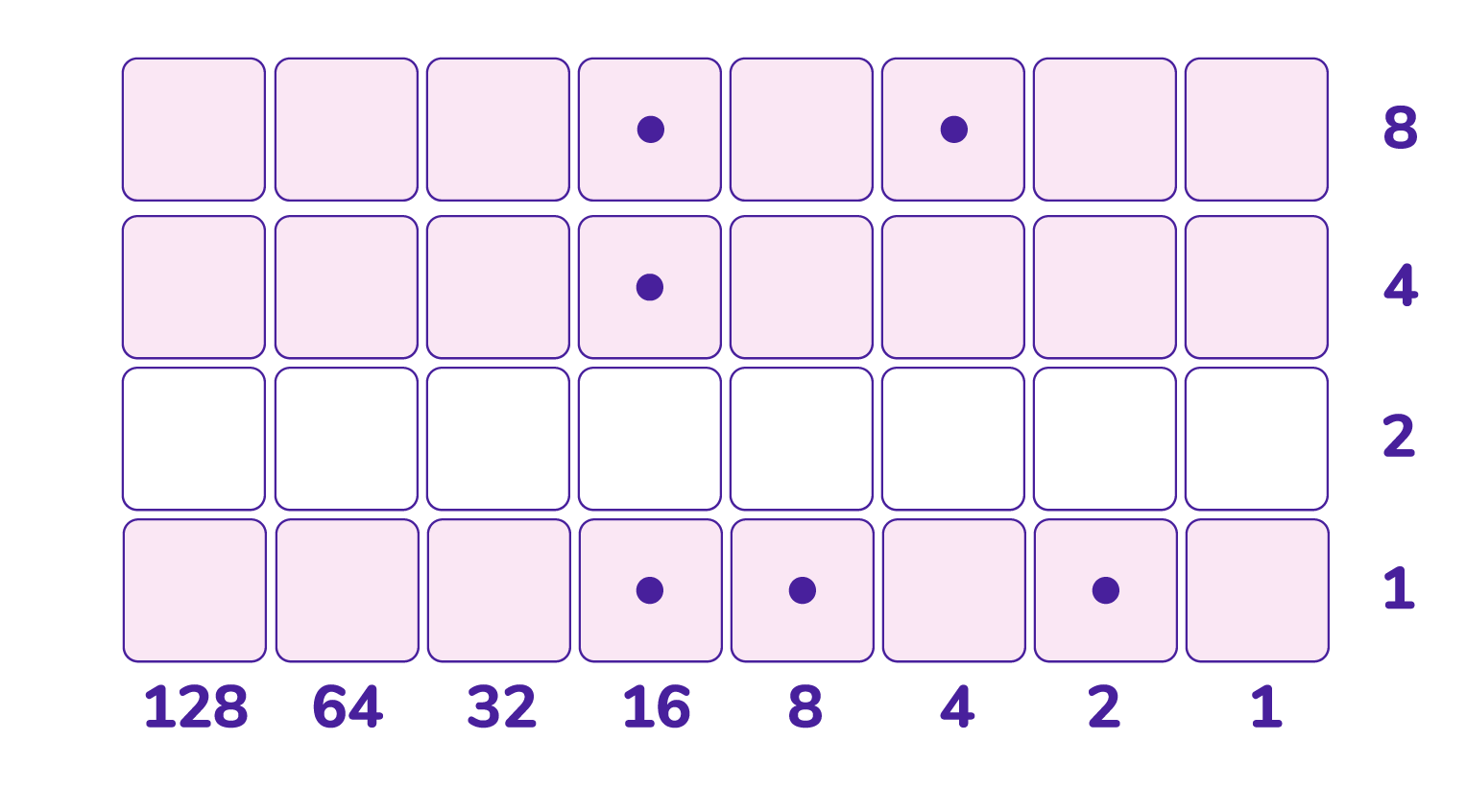What’s the leftmost column we can complete right now without ever touching those dost of the $16$s column? We see that there is no means complete the $8$s column. (What dot can we slide into its top?)

There is no means to complete the $4$s column either. (How do we slide a dot into that $4 \times 4$ cell?)

So let’s work on the $2$s column. I can see by sliding the dot in the $8$s column and performing a (horizontal) unexplosion from the $4$s column we can fill up the $2$s column.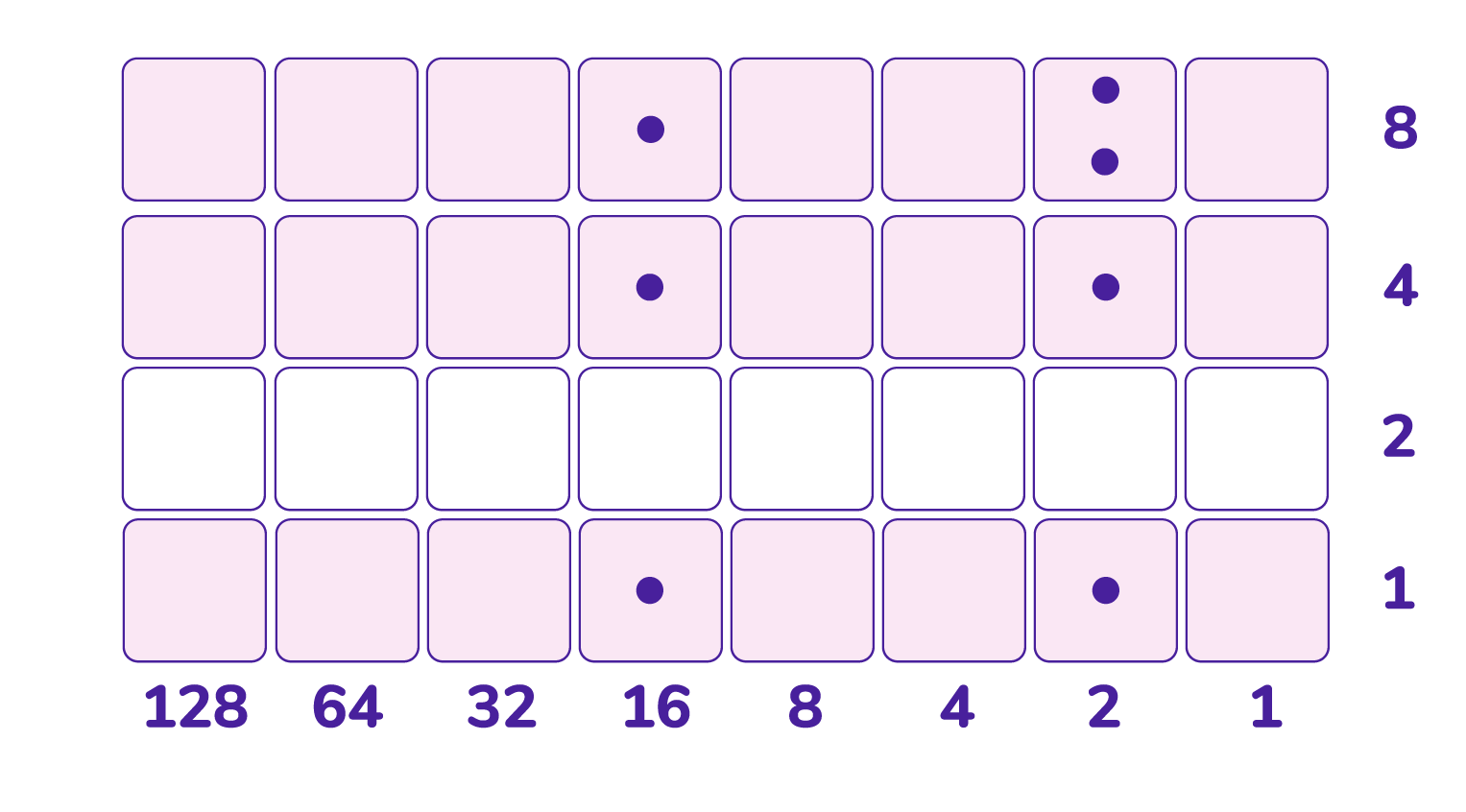The $2$s column is a bit overloaded. Let’s unexplode one of the dots the top pair (horizontally).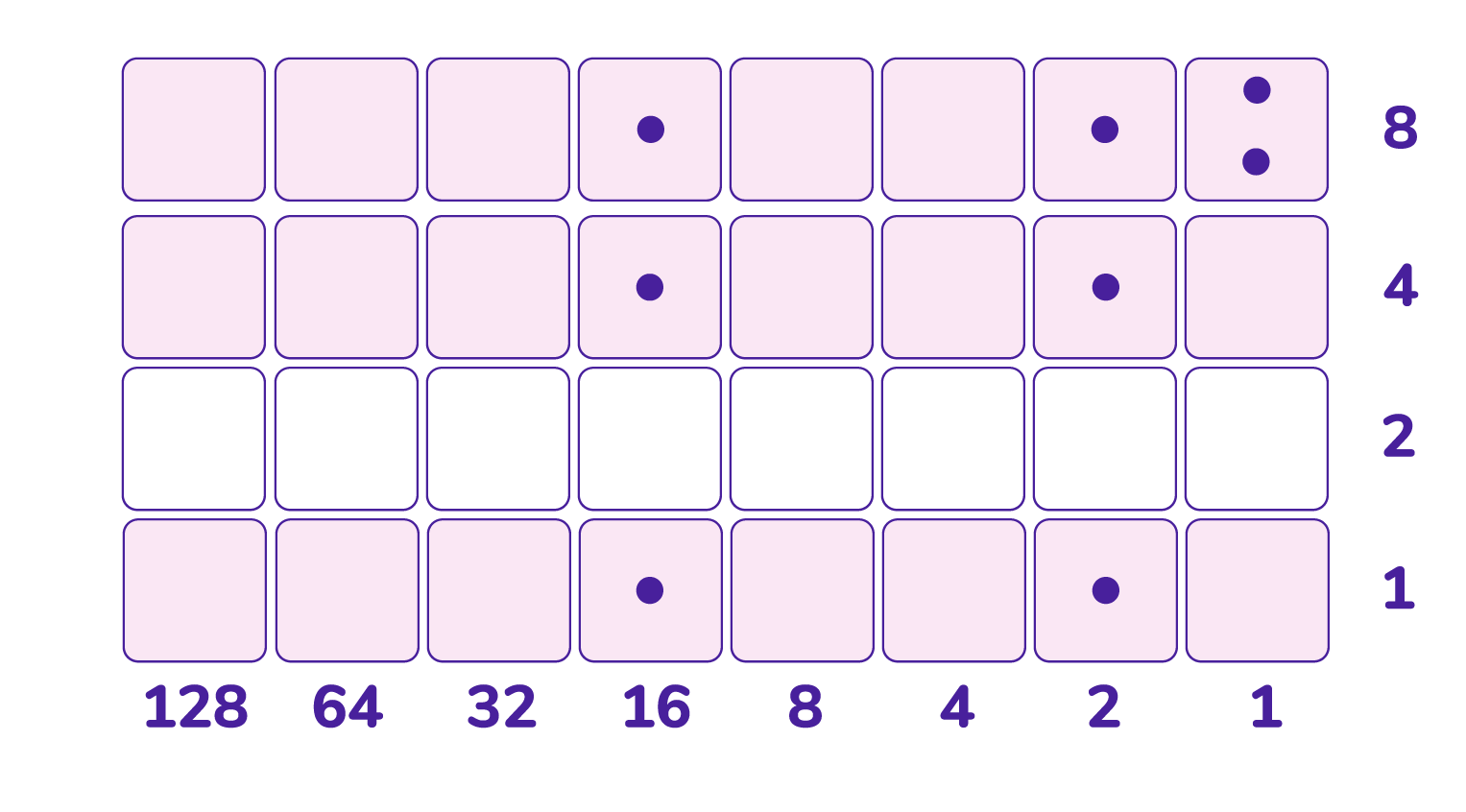All the action is now left in the $1$s column. What can we do to make that column complete? (Remember, dots in completed columns are never to be touched again.) Let’s unexplode downwards a number of times.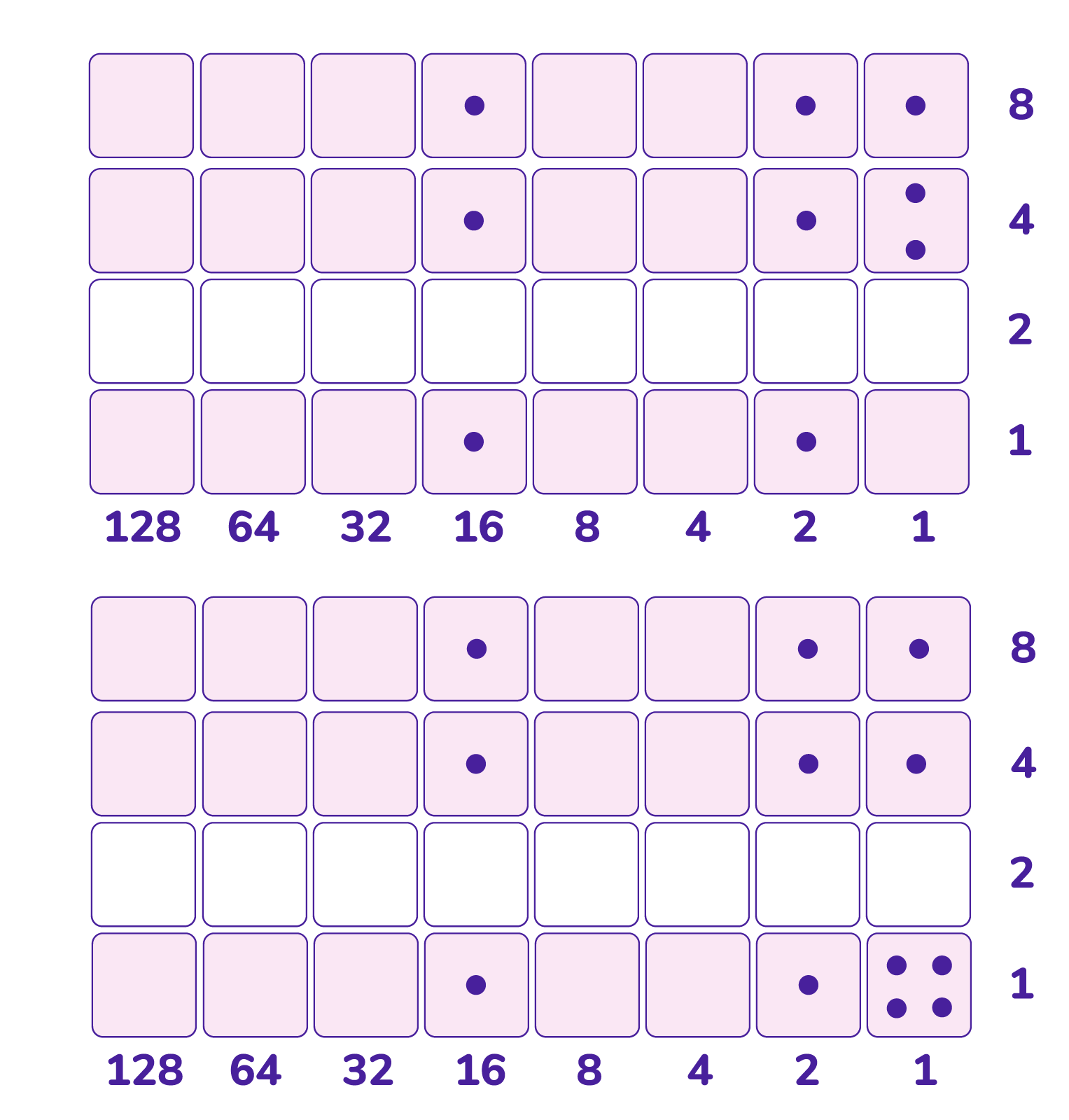This does complete the $1$s column, but with three ones too many.

If we had three less dots —$247$ instead of $250$— then we would have, right now, a picture of $19 \times 13$ showing that $247 \div 13 = 19$. So it must be then that $250 \div 13$ has a remainder of three and so

$250 \div 13 = 19 + \dfrac{3}{13}$.

Question: Compute $256 \div 10$ via Napier’s method.

Question: Is it possible to do polynomial division with Napier’s checkerboard? (Can one compute $\dfrac{1}{1-x}$?)